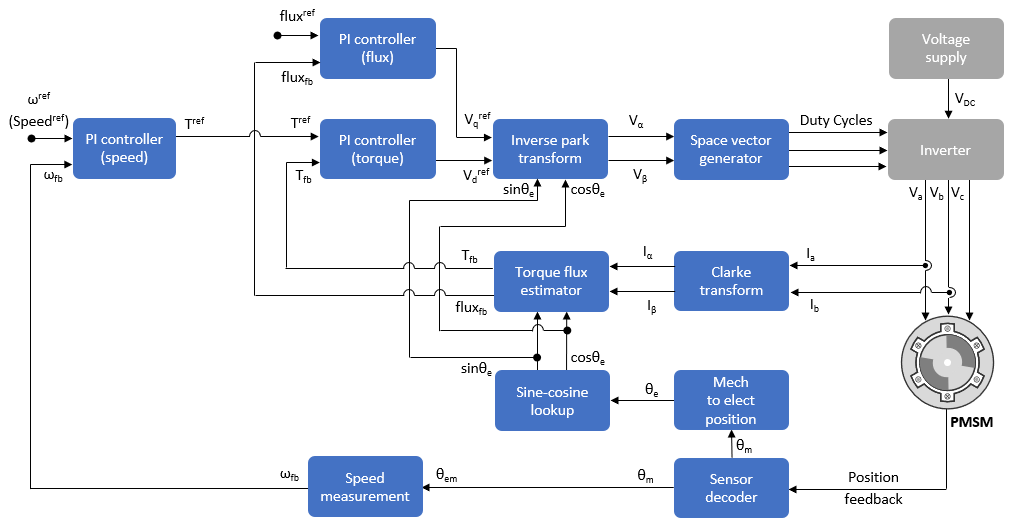## Direct Torque Control (DTC)

Direct Torque Control (DTC) is a vector motor control technique that implements motor speed control by directly controlling the flux and torque of the motor. Unlike field-oriented control (FOC) that controls d- and q-axis motor currents, the DTC algorithm estimates the torque and flux values from the motor position and currents. Then it uses PI controllers to control the motor torque and flux to eventually generate the optimum voltages that run the motor.

Motor Control Blockset™ uses the DTC space vector pulse-width modulation (DTC-SVPWM) variant to control a permanent magnet synchronous motor (PMSM). The technique uses space vector modulation (SVM) to produce the pulse-width modulation (PWM) duty cycles that are used by the inverter to generate the three-phase voltages that run the PMSM.

The DTC-SVPWM algorithm estimates the motor torque and flux feedback values using the current feedback (in the α-β reference frame) from the motor. The algorithm uses the motor speed feedback to compute the flux reference value. The speed PI controller (part of the outer control loop) uses the speed error input to compute the torque reference value. The flux and torque PI controllers (part of the inner control loop) use these flux and torque references and flux and torque feedback values to compute the d-axis and q-axis reference voltages. The algorithm uses the PWM Reference Generator block to generate PWM duty-cycles (using SVM) from these reference voltages.

You can determine current rotor position using both sensor-based or sensorless approaches.### Flux and Torque Estimation

The DTC-SVPWM algorithm used by Motor Control Blockset uses these transient machine model equations to estimate flux and torque of a PMSM.

These equations describe flux estimation from the currents in the α-β reference frame and rotor position:

`${\psi }_{\alpha }={L}_{s}\cdot {i}_{\alpha }+{\psi }_{PM}\cdot \mathrm{cos}\theta$`
`${\psi }_{\beta }={L}_{s}\cdot {i}_{\beta }+{\psi }_{PM}\cdot \mathrm{sin}\theta$`
`$\psi =\sqrt{{\psi }_{\alpha }^{2}+{\psi }_{\beta }^{2}}$`

These equations describe the per-unit (PU) computation of flux:

`${\psi }_{\alpha }^{pu}=\left({\omega }_{base}\cdot {L}_{s}^{pu}\cdot {i}_{\alpha }^{pu}\right)+\left({\psi }_{PM}^{pu}\cdot \mathrm{cos}\theta \right)$`
`${\psi }_{\beta }^{pu}=\left({\omega }_{base}\cdot {L}_{s}^{pu}\cdot {i}_{\beta }^{pu}\right)+\left({\psi }_{PM}^{pu}\cdot \mathrm{sin}\theta \right)$`
`${\psi }^{pu}=\sqrt{{\left({\psi }_{\alpha }^{pu}\right)}^{2}+{\left({\psi }_{\beta }^{pu}\right)}^{2}}$`
`${\omega }_{base}=2\cdot \pi \cdot {f}_{base}$`

These equations describe torque estimation from the currents in the α-β reference frame:

`$T=\frac{3}{2}\cdot p\cdot \left({\psi }_{\alpha }{i}_{\beta }-{\psi }_{\beta }{i}_{\alpha }\right)$`

These equations describe the per-unit (PU) computation of torque:

`${T}^{pu}=\frac{1}{{\psi }_{PM}^{pu}}\cdot \left({\psi }_{\alpha }^{pu}{i}_{\beta }^{pu}-{\psi }_{\beta }^{pu}{i}_{\alpha }^{pu}\right)$`

where:

• ψ is the rotor flux of PMSM (Weber).

• ψpu is the per-unit version of ψ (Weber).

• ψα is the rotor flux along the α-axis of the α-β reference frame (Weber).

• ψαpu is the per-unit version of ψα (Weber).

• ψβ is the rotor flux along the β-axis of the α-β reference frame (Weber).

• ψβpu is the per-unit version of ψβ (Weber).

• ψPM is the permanent magnet flux linkage of the PMSM (Weber).

• ψPMpu is the per-unit version of ψPM (Weber).

• Ls is the stator inductance of the PMSM (Henry).

• Lspu is the per-unit version of Ls (Henry).

• iα is the motor current along the α-axis of the α-β reference frame (Amperes).

• iαpu is the per-unit version of iα (Amperes).

• iβ is the motor current along the β-axis of the α-β reference frame (Amperes).

• iβpu is the per-unit version of iβ (Amperes).

• θ is the rotor position (captured by a sensor or determined by sensorless position estimation) (Radians).

• ωbase is the mechanical base speed of the motor (Radians/ sec).

• fbase is the mechanical frequency of the motor (Hertz).

• T is the rotor torque (Nm).

• Tpu is the per-unit version of T (Nm).

• p is the number of pole pairs of the motor.